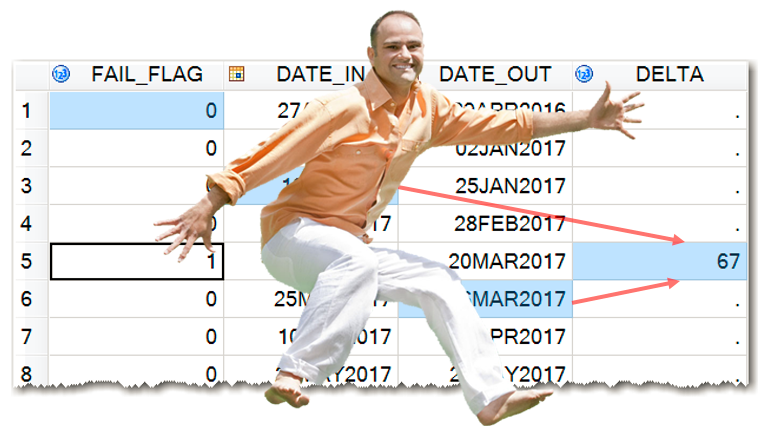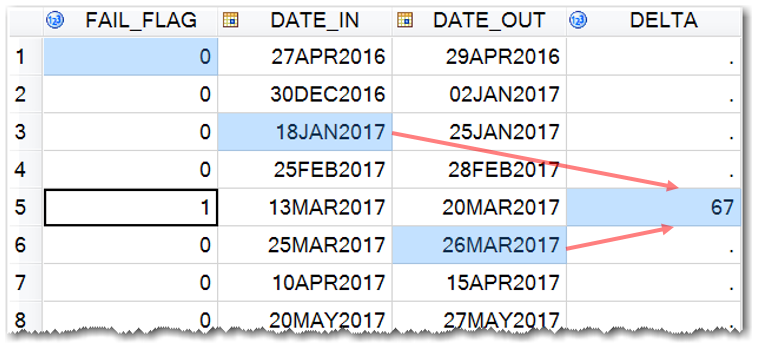The purpose of this blog post is to demonstrate a SAS coding technique that allows for calculations with multiple variables across a SAS dataset, whether or not their values belong to the same or different observations.### What do we want?

As illustrated in the picture on the right, we want to be able to hop, or jump, back and forth, up and down across observations of a data table in order to implement calculations not just on different variables, but with their values from different observations of a data table.

In essence, we want to access SAS dataset variable values similar to accessing elements of a matrix (aij), where rows represent dataset observations, and columns represent dataset variables.

### Combine and Conquer

In the spirit of my earlier post Combine and Conquer with SAS, this technique combines the functionality of the LAG function, which allows us to retrieve the variable value of a previous observation from a queue, with an imaginary, non-existent in SAS, LEAD function that reads a subsequent observation in a data set while processing the current observation during the same iteration of the DATA step.

### LAG function

LAG<n> function in SAS is not your usual, ordinary function. While it provides a mechanism of retrieving previous observations in a data table, it does not work “on demand” to arbitrarily read a variable value from a previous observation n steps back. If you want to use it conditionally in some observations of a data step, you still need to call it in every iteration of that data step. That is because it retrieves values from a queue that is built sequentially for each invocation of the LAG<n> function. In essence, in order to use the LAG function even just once in a data step, you need to call it every time in each data step iteration until that single use.

Moreover, if you need to use each of the LAG1, LAG2, . . . LAGn functions just once, in order to build these queues, you have to call each of them in every data step iteration even if you are going to use them in some subsequent iterations.

The LEAD function is implemented in Oracle SQL and it returns data from the next or subsequent row of a data table. It allows you to query more than one row in a table at a time without having to join the table to itself.

There is no such function in SAS. However, the POINT= option of the SET statement in a SAS data step allows retrieving any observation by its number from a data set using random (direct) access to read a SAS data set. This will allow us to simulate a LEAD function in SAS.

### HOP function

But why do we need two separate functions like LAG and LEAD in order to retrieve non-current observations. In essence, these two functions do the same thing, just in opposite directions. Why can’t we get by with just one function that does both backwards and forwards “hopping?”

Let’s combine and conquer.

Ideally, we would like to construct a new single function - let’s call it HOP(x, j) - that combines the best qualities of both LAG and LEAD functions. The two arguments of the HOP function would be as follows:

x – SAS variable name (numeric or character) the value of we are retrieving;

j – hop distance (numeric) – an offset from the current observation; negative values being lagging (hopping back), positive values being leading (hopping forward), and a zero-value meaning staying within the current observation.

The sign of the second argument defines whether we lag (minus) or lead (plus). The absolute value of this second argument defines how far from the current observation we hop.

Alternatively, we could have the first argument as a column number, and the second argument as a row/observation number, if we wanted this function to deal with the data table more like with a matrix. But relatively speaking, the method doesn’t really matter as long as we can unambiguously identify a data element or a cell. To stay within the data step paradigm, we will stick with the variable name and offset from the current observation (_n_) as arguments.

Let’s say we have a data table SAMPLE, where for each event FAIL_FLAG=1 we want to calculate DELTA as the difference between DATE_OUT, one observation after the event, and DATE_IN, two observations before the event:That is, we want to calculate DELTA in the observation where FAIL_FLAG = 1 as

26MAR2017 18JAN2017 = 67 (as shown in light-blue highlighting in the above figure).

With the HOP() function, that calculation in the data step would look like this:

```data SAMPLE; set SAMPLE; if FAIL_FLAG then DELTA = hop(DATE_OUT,1) - hop(DATE_IN,-2); run;```

It would be reasonable to suggest that the hop() function should return a missing value when the second argument produces an observation number outside of the dataset boundary, that is when

_n_ + j < 0 or _n_ + j > num, where _n_ is the current observation number of the data step iteration; num is the number of observations in the dataset; j is the offset argument value.

Do you see anything wrong with this solution? I don’t. Except that the HOP function exists only in my imagination. Hopefully, it will be implemented soon if enough SAS users ask for it. But until then, we can use its surrogate in the form of a %HOP macro.

### %HOP macro

The HOP macro grabs the value of a specified variable in an offset observation relative to the current observation and assigns it to another variable. It is used within a SAS data step, but it cannot be used in an expression; each invocation of the HOP macro can only grab one value of a variable across observations and assign it to another variable within the current observation.

If you need to build an expression to do calculations with several variables from various observations, you would need to first retrieve all those values by invoking the %hop macro as many times as the number of the values involved in the expression.

Here is the syntax of the %HOP macro, which has four required parameters:

%HOP(d,x,y,j)

d – input data table name;

x – source variable name;

y – target variable name;

j – integer offset relative to the current observation. As before, a negative value means a previous observation, a positive value means a subsequent observation, and zero means the current observation.

Using this %HOP macro we can rewrite our code for calculating DELTA as follows:

``` data SAMPLE (drop=TEMP1 TEMP2); set SAMPLE; if FAIL_FLAG then do; %hop(SAMPLE,DATE_OUT,TEMP1, 1) %hop(SAMPLE,DATE_IN, TEMP2,-2) DELTA = TEMP1 - TEMP2; end; run;```

Note that we should not have temporary variables TEMP1 and TEMP2 listed in a RETAIN statement, as this could mess up our calculations if the j-offset throws an observation number out of the dataset boundary.

Also, the input data table name (d parameter value) is the one that is specified in the SET statement, which may or may not be the same as the name specified in the DATA statement.

In case you are wondering where you can download the %HOP macro from, here it is in its entirety:

```%macro hop(d,x,y,j); _p_ = _n_ + &j; if (1 le _p_ le _o_) then set &d(keep=&x rename=(&x=&y)) point=_p_ nobs=_o_; %mend hop;```

Of course, it is “free of charge” and “as is” for your unlimited use.